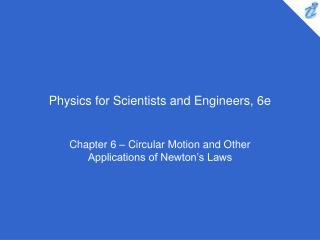# Physics for Scientists and Engineers, 6e - PowerPoint PPT PresentationDownload PresentationPhysics for Scientists and Engineers, 6e

Physics for Scientists and Engineers, 6e
Download Presentation## Physics for Scientists and Engineers, 6e

- - - - - - - - - - - - - - - - - - - - - - - - - - - E N D - - - - - - - - - - - - - - - - - - - - - - - - - - -
##### Presentation Transcript

1. Physics for Scientists and Engineers, 6e Chapter 6 – Circular Motion and Other Applications of Newton’s Laws

2. You are riding on a Ferris wheel that is rotating with constant speed. The car in which you are riding always maintains its correct upward orientation - it does not invert. What is the direction of your centripetal acceleration when you are at the top of the wheel? • upward • downward • impossible to determine

3. The centripetal acceleration is always toward the center of the circular path.

4. You are riding on the Ferris wheel of question 1. What is the direction of the normal force exerted by the seat on you when you are at the top of the wheel? • upward • downward • impossible to determine

5. The normal force is always perpendicular to the surface that applies the force. Because your car maintains its orientation at all points on the ride, the normal force is always upward.

6. Which of the following is impossible for a car moving in a circular path? • the car has tangential acceleration but no centripetal acceleration. • the car has centripetal acceleration but no tangential acceleration. • the car has both centripetal acceleration and tangential acceleration.

7. If the car is moving in a circular path, it must have centripetal acceleration given by Equation 4.15.

8. Consider the passenger in the car making a left turn in the figure below. Which of the following is correct about forces in the horizontal direction if the person is making contact with the right-hand door? • The passenger is in equilibrium between real forces acting to the right and real forces acting to the left. • The passenger is subject only to real forces acting to the right. • The passenger is subject only to real forces acting to the left. • None of these is true.

9. The only forces acting on the passenger are the contact force with the door and the friction force from the seat. Both of these are real forces and both act to the left in Figure 6.11. Fictitious forces should never be drawn in a force diagram.

10. A baseball and a basketball, having the same mass, are dropped through air from rest such that their bottoms are initially at the same height above the ground, on the order of 1 m or more. Which one strikes the ground first? • the baseball • the basketball • both strike the ground at the same time

11. The basketball, having a larger cross-sectional area, will have a larger force due to air resistance than the baseball. This will result in a smaller net force in the downward direction and a smaller downward acceleration.